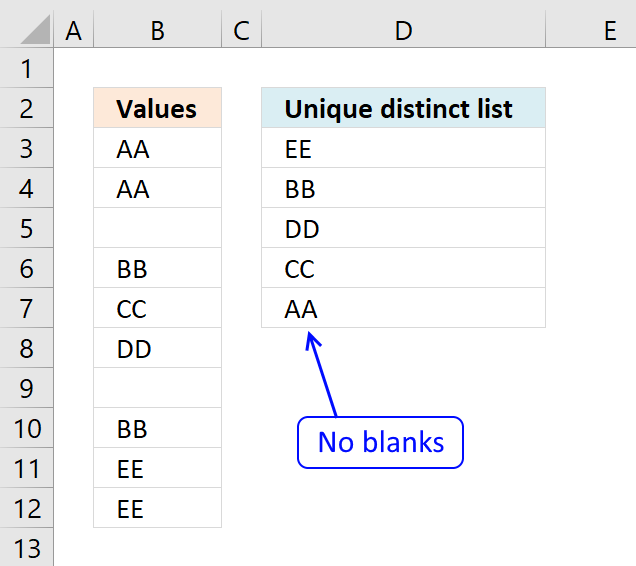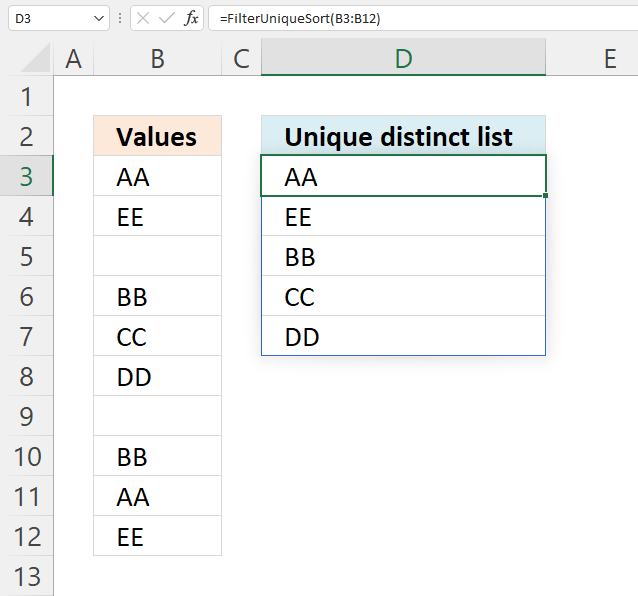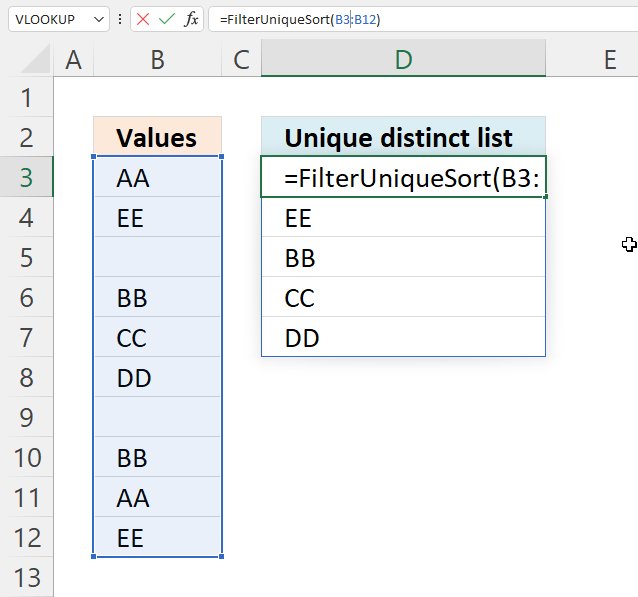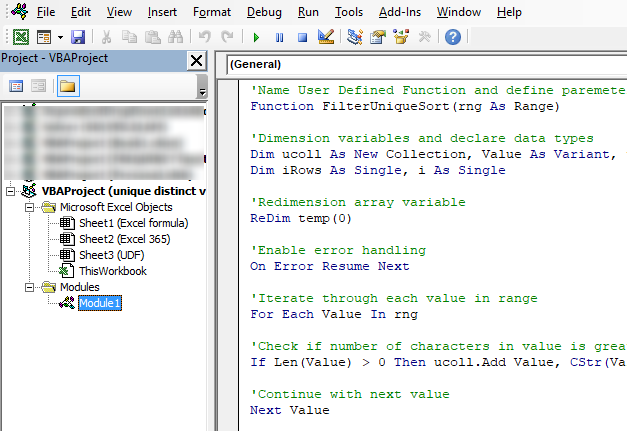Author: Oscar Cronquist Article last updated on September 15, 2021This article demonstrates formulas that extract unique distinct values and ignore blank empty cells.

## 1. Extract a unique distinct list and ignore blanksQuestion: How do I extract a unique distinct list from a column containing blanks?

Answer: Cell range B3:B12 contains several blank cells. The following formula in cell D3 extracts unique distinct values from cell range B3:B12. Unique distinct values are all values except duplicates are merged into one distinct value.

Formula in D3:

=LOOKUP(2, 1/((COUNTIF(\$D\$2:D2, \$B\$3:\$B\$12)=0)*(\$B\$3:\$B\$12<>"")), \$B\$3:\$B\$12)

Copy cell B2 and paste to cells below.

### 1.1 Explaining the LOOKUP formula in cell D3

#### Step 1 - Check cell range B3:B12 for non-empty cells

If a cell contains a value TRUE is returned. The following line is a logical expression, cells not equal to nothing return TRUE. The less and larger than characters are logical operators that evaluates to boolean values, True or False.

\$B\$3:\$B\$12<>""

becomes

{"AA";"AA";0;"BB";"CC";"DD";0;"BB";"EE";"EE"}<>""

and returns

{TRUE;TRUE; FALSE;TRUE; TRUE;TRUE; FALSE;TRUE; TRUE;TRUE}

#### Step 2 - Ignore duplicate cells

The COUNTIF function counts cells that equal a condition or any of the supplied criteria. The first argument has both an absolute and relative cell reference. This allows the cell range to grow when cell B3 is copied to cells below as far as needed.

COUNTIF(\$D\$2:D2, \$B\$3:\$B\$12)=0

becomes

COUNTIF("Unique distinct list",{"AA";"AA";0;"BB";"CC";"DD";0;"BB";"EE";"EE"})=0

becomes

{0;0;0;0;0;0;0;0;0;0}=0

The equal sign is also a logical operator like the less and greater signs, it evaluates tor True or False.

{0;0;0;0;0;0;0;0;0;0}=0

and returns

{TRUE; TRUE; TRUE; TRUE; TRUE; TRUE; TRUE; TRUE; TRUE; TRUE}

How to use the COUNTIF function

Counts the number of cells that meet a specific condition.

#### Step 3 - Multiply arrays

Multiplying boolean values is the same as applying OR logic to each value based on their position.

The first value in the first array is True and in the second array is also True. True * True equals 1.

The other possibilties are:

• True * False = 0 (zero)
• False * True = 0 (zero)
• False * False = 0 (zero)

The boolean equivalent to True is 1 and False is 0 (zero).

(COUNTIF(\$D\$2:D2, \$B\$3:\$B\$12)=0)*(\$B\$3:\$B\$12<>"")

becomes

{TRUE; TRUE; TRUE; TRUE; TRUE; TRUE; TRUE; TRUE; TRUE; TRUE}* {TRUE;TRUE; FALSE;TRUE; TRUE;TRUE; FALSE;TRUE; TRUE;TRUE}

and returns

{1; 1; 0; 1; 1; 1; 0; 1; 1; 1}

#### Step 4 - Divide 1 by the array

The reason I am dividing 1 with the array is to replace 0 (zero) with the #DIV/0. The LOOKUP function will ignore the #DIV/0 errors, shown in the next step.

1/((COUNTIF(\$D\$2:D2, \$B\$3:\$B\$12)=0)*(\$B\$3:\$B\$12<>""))

becomes

1/({1; 1; 0; 1; 1; 1; 0; 1; 1; 1})

and returns

{1; 1; #DIV/0!; 1; 1; 1; #DIV/0!; 1; 1; 1}

#### Step 5 - Find last match in array and return corresponding value

LOOKUP(2, 1/((COUNTIF(\$D\$2:D2, \$B\$3:\$B\$12)=0)*(\$B\$3:\$B\$12<>"")), \$B\$3:\$B\$12)

becomes

LOOKUP(2, {1;1;#DIV/0!;1;1;1;#DIV/0!;1;1;1}, \$B\$3:\$B\$12)

becomes

LOOKUP(2, {1;1;#DIV/0!;1;1;1;#DIV/0!;1;1;1}, {"AA";"AA"; 0;"BB";"CC"; "DD";0;"BB"; "EE";"EE"})

and returns "EE" in cell D3.

## 2. Extract a unique distinct list and ignore blanksUpdate 10th December 2020: Excel 365 subscribers can now use this regular formula in cell D3.

=UNIQUE(FILTER(B3:B12,B3:B12<>""))

There is no need to use absolute cell references with formulas that return a dynamic array, however, it is crucial that you use them with the first formula above, as shown.

Note that the formula above deploys an array of values to the appropriate cell range. If any of the cells below are populated cell D3 returns a #SPILL! error.

Check out how the Excel 365 formula works here:
Extract unique distinct values ignoring blanks

Recommended articles
FILTER function | UNIQUE function | LET function | XMATCH function | XLOOKUP function | Excel Function Library

## 3. Extract a unique distinct list and ignore blanks (UDF)The image above shows a User Defined Function that extracts unique distinct values from a specific cell range.

What is a unique distinct value?
Unique distinct values are all values, however, duplicate values are merged into one distinct value. In other words, there are no duplicate values in the extracted list.

What is a User Defined Function (UDF)?
A UDF is a custom function that you can create yourself using Visual Basic for Applications (VBA) code. The code must be inserted into a code module in your workbook before you can use the custom function.

Formula in cell D3:

=FilterUniqueSort(B3:B12)

### 3.1 User Defined Function Syntax

FilterUniqueSort(rng)

### 3.2 User Defined Function argumentsrng - A reference to a cell range you want to extract values from. The example above uses cell reference B3:B12.

### 3.3 VBA code

```'Name User Defined Function and define paremeter
Function FilterUniqueSort(rng As Range)

'Dimension variables and declare data types
Dim ucoll As New Collection, Value As Variant, temp() As Variant
Dim iRows As Single, i As Single

'Redimension array variable
ReDim temp(0)

'Enable error handling
On Error Resume Next

'Iterate through each value in range
For Each Value In rng

'Check if number of characters in value is greater than 0 (zero), if true add value to collection ucoll
If Len(Value) > 0 Then ucoll.Add Value, CStr(Value)

'Continue with next value
Next Value

'Disable error handling
On Error GoTo 0

'Iterate through each value in collection ucoll
For Each Value In ucoll

'Save value to last container in array variable temp
temp(UBound(temp)) = Value

'Add new container to array variable temp
ReDim Preserve temp(UBound(temp) + 1)

'Next value
Next Value

'Remove last container in array variable temp
ReDim Preserve temp(UBound(temp) - 1)

'Transpose values in array variable temp and return those values to worksheet
FilterUniqueSort = Application.Transpose(temp)

End Function
```

### 3.4 Where to put the code?1. Press shortcut keys Alt + F11 to open the Visual Basic Editor (VBE).
2. Press the left mouse button on "Insert" on the menu, see the image above. A pop-up menu appears.
3. Press the left mouse button on "Module" to create a module to your workbook.
4. Copy (Ctrl + c) above VBA code
5. Paste (Ctrl +v)  to the code module, see the image above.